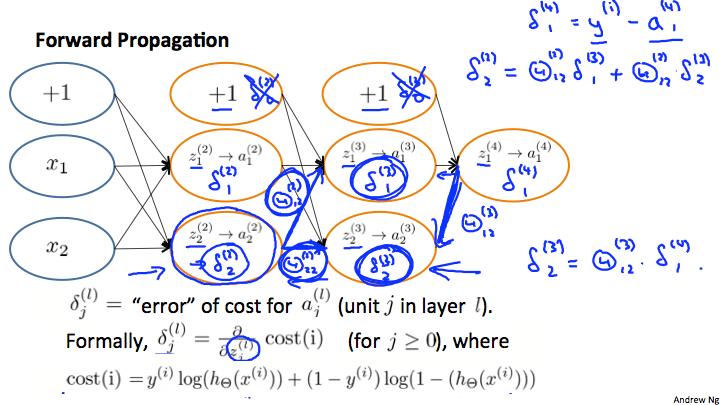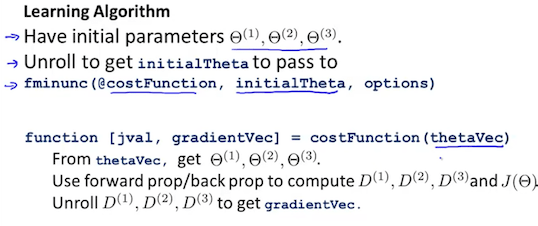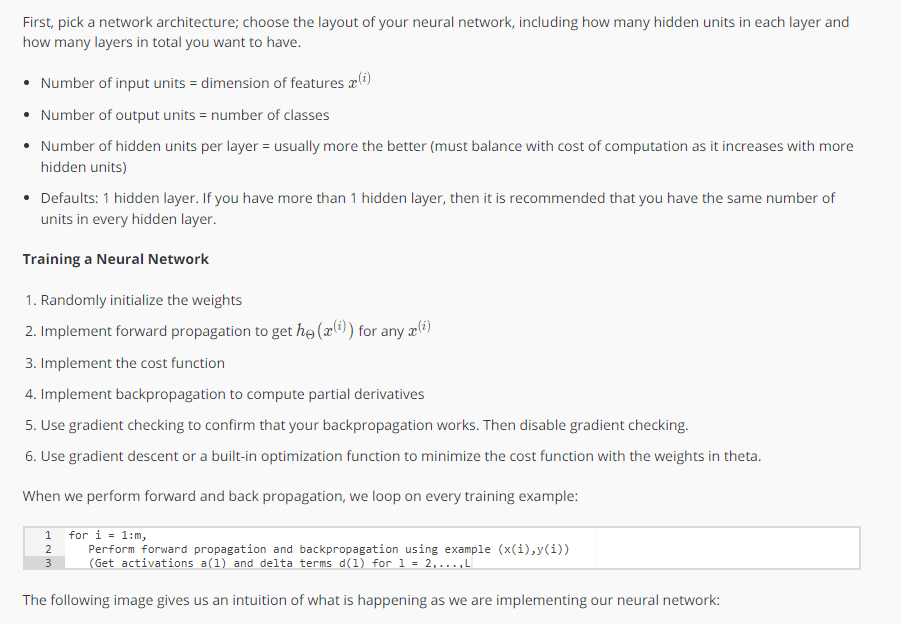吴恩达机器学习笔记-反向传播算法练习

直观感受反向传播的概念\delta_j^(l) = \frac{\partial}{\partial z_j^(l)}cost(t)\delta_2^(2) = \Theta_{12}^(2)\delta_1^(3) + \Theta_{12}^(2)\delta_1^(3)
\delta_2^(3) = \Theta_{12}^(3)\delta_1^(4)

参数展开

thetaVector = [Theta1(:);Theta2(:),Theta(3);]

Theta1 = reshape(thetaVector(1:110),10_11) \\ Theta2 = reshape(thetaVector(111:220),10_11) \\ Theta3 = reshape(thetaVector(221:231),1_11) \\梯度校验

\frac{\partial}{\partial \Theta}J(\Theta) \approx \frac{J(\Theta+\epsilon) - J(\Theta - \epsilon)}{2\epsilon}

\frac{\partial}{\partial \Theta}J(\Theta) \approx \frac{J(\Theta_1,... \Theta_j+\epsilon,...,\Theta_n) - J(\Theta_1...,\Theta_j - \epsilon,... \Theta_n)}{2\epsilon}随机初始化参数Theta1 = rand(10,11) * (2*INIT_EPSILON) - INIT_EPSILON;
Theta2 = rand(10,11) * (2*INIT_EPSILON) - INIT_EPSILON;
Theta3 = rand(1,11) * (2*INIT_EPSILON) - INIT_EPSILON;

总结